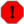## pvsmooth

pvsmooth — Smooth the amplitude and frequency time functions of a pv stream using parallel 1st order lowpass IIR filters with time-varying cutoff frequency.

## Description

Smooth the amplitude and frequency time functions of a pv stream using a 1st order lowpass IIR with time-varying cutoff frequency. This opcode uses the same filter as the tone opcode, but this time acting separately on the amplitude and frequency time functions that make up a pv stream. The cutoff frequency parameter runs at the control-rate, but unlike tone and tonek, it is not specified in Hz, but as fractions of 1/2 frame-rate (actually the pv stream sampling rate), which is easier to understand. This means that the highest cutoff frequency is 1 and the lowest 0; the lower the frequency the smoother the functions and more pronounced the effect will be.

These are filters applied to control signals so the effect is basically blurring the spectral evolution. The effects produced are more or less similar to pvsblur, but with two important differences: 1.smoothing of amplitudes and frequencies use separate sets of filters; and 2. there is no increase in computational cost when higher amounts of 'blurring' (smoothing) are desired.

## Syntax

`fsig pvsmooth fsigin, kacf, kfcf`

## Performance

fsig -- output pv stream

fsigin -- input pv stream.

kacf -- amount of cutoff frequency for amplitude function filtering, between 0 and 1, in fractions of 1/2 frame-rate.

kfcf -- amount of cutoff frequency for frequency function filtering, between 0 and 1, in fractions of 1/2 frame-rate.

WarningIt is unsafe to use the same f-variable for both input and output of pvs opcodes. Using the same one might lead to undefined behavior on some opcodes. Use a different one on the left and right sides of the opcode.

## Examples

Here is an example of the pvsmooth opcode. It uses the file pvsmooth.csd.

Example 849. Example of the pvsmooth opcode.

See the sections Real-time Audio and Command Line Flags for more information on using command line flags.

```<CsoundSynthesizer>
<CsOptions>
; Select audio/midi flags here according to platform
-odac  ;;;realtime audio out
; For Non-realtime ouput leave only the line below:
; -o pvsmooth.wav -W ;;; for file output any platform
</CsOptions>
<CsInstruments>

sr = 44100
ksmps = 32
nchnls = 2
0dbfs  = 1

instr 1

kacf = p4
kfcf = p5
asig soundin "fox.wav"
fsig pvsanal asig, 1024, 256, 1024, 1	; analyse it
ftps pvsmooth fsig, kacf, kfcf
atps pvsynth ftps			; synthesise it
outs atps*3, atps*3

endin
</CsInstruments>
<CsScore>
;       amp  freq
i 1 0 3 0.01 0.01	;smooth amplitude and frequency with cutoff frequency of filter at 1% of 1/2 frame-rate (ca 0.86 Hz)
i 1 + 3  1   0.01	;no smoothing on amplitude, but frequency with cf at 1% of 1/2 frame-rate (ca 0.86 Hz)
i 1 + 10 .001  1	;smooth amplitude with cf at 0.1% of 1/2 frame-rate (ca 0.086 Hz)
;and no smoothing of frequency
e
</CsScore>
</CsoundSynthesizer>
```

The formula for calculating the cutoff frequency of the filter: frame rate / hopsize = new frame starts per second (in Hz), then the percentage of half the framerate. For example, looking at the first note in the example, the frame rate is 44100 / 256 = 172,265625 Hz (= 172 new frame starts per second). half of the frame rate is about 86 Hz, and one percent of this is 0.86 Hz.

## Credits

 Author: Victor Lazzarini May 2006

New plugin in version 5

May 2006.